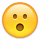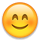# Footer Callback for Multiple Columns

Dear Sirs

How can we have pageTotal for multiple columns, for example if there are numerical data at (4th, 5th, 6th columns) all need a pageTotal. how will the below script be amended ?

"footerCallback": function ( row, data, start, end, display ) {
var api = this.api(), data;

``````        // Remove the formatting to get integer data for summation
var intVal = function ( i ) {
return typeof i === 'string' ?
i.replace(/[\\$,]/g, '')*1 :
typeof i === 'number' ?
i : 0;
};

// Total over all pages
total = api
.column( 4 )
.data()
.reduce( function (a, b) {
return intVal(a) + intVal(b);
}, 0 );

pageTotal = api
.column( 4, { page: 'current'} )
.data()
.reduce( function (a, b) {
return intVal(a) + intVal(b);
}, 0 );

// Update footer
jQuery( api.column( 4 ).footer() ).html(
// '\$'+pageTotal +' ( \$'+ total +' total)'
pageTotal.toFixed( 2 )
);
},
``````

• I would use a for loop to iterate an array of columns and put the `total`, `pageTotal` and `Update footer` code in the loop. You would then change the column number from 4 to the element in the array of the for loop.

Kevin

• Its just as Kevin says - loop over the columns that you want to do the sum on. I've put together this little example that uses `columns().every()` to select the columns which have a class of `sum` and then sums them.

Regards,
Allan

• @allan: nice solution!

What now if I want to keep (for each column) the sum of all pages AND the sum of the current page? A sort of TOTAL/CURRENT sum for each column.

I don't know within the .every function how to filter out the column that aren't on the current page.

Any clues?
Thanks

• edited May 2018

Here's the code I have:

``````var dataTableColumnSum = function () {
var api = this.api();

api.columns('.footerSumEnabled').every(function () {
var elem = \$(this);
console.log(elem);
var total = this
.data()
.reduce(function (a, b) {
return ParseNumber(a) + ParseNumber(b);
}, 0);

var pageTotal = this
//.column(indexColumn, { page: 'current' })
.data()
.reduce(function (a, b) {
return ParseNumber(a) + ParseNumber(b);
}, 0);

\$(this.footer()).html(
FormatDataTableNumber(pageTotal) + " (" + FormatDataTableNumber(total) + " totali)"
);
});
}
``````

But as you can see, it show the same sum values for both total and pageTotal.

• Are you using server-side processing by any chance? If so, that's the issue, since only the data for the current page is available at the client-side.

Allan

• edited May 2018

But that's workingMy problem is different. I would like to differentiate totals per page/total, as shown here , but on multiple columns, not only one.

I'm filtering client side! I've such as 100 records, and I show 10 per page (so 10 pages).
So, if the total of column X is 1000 and total of column X is 10000 (in total), I'd like to show "N (1000 total)" and "M (10000 total)", not just 1000 and 10000.

Is it more clear now?

• So the issue is that you just need to know how to address the second row in the footer so you can write a value into it? You have the value already?

Use `\$('#myTable tfoot tr').eq(1).find('th').eq(columnIndex).html( ... );` updating the table name and column index as needed of course.

Allan

• edited May 2018

No my issue is that I don't know how to sum (by the reduce() function) the values from the current pageI'm able to catch only the total sum, not the current page one.

Look: using .every() there isn't anymore .column(indexColumn, { page: 'current' }).
I can use it prior to .every(), but than I'll miss the total amount.

• The example you linked to shows how to do that. If that isn't working for you, could you link to your page or show me your code please?

Thanks,
Allan

• It show how to do with 1 column. My request is with mutiple columns (which is why I use .every())There's where I'm not sure how to do it... lol

• OOHH! I see. Could you give me a link to your page showing the issue please? The code above looks like it should be working.

Allan

• It works, but for both (var total and var pageTotal) it does the same sum, because its the same code!

I don't know how to differentiate pageTotal from total, in a way that the former will only sum the column of the current page, not all page (as total does)• I'm with you now - sorry for being a bit slow on this one!

``````var dataTableColumnSum = function () {
var api = this.api();

api.columns('.footerSumEnabled').every(function () {
var elem = \$(this);

var total = api
.column( this.index() )
.data()
.reduce(function (a, b) {
return ParseNumber(a) + ParseNumber(b);
}, 0);

var pageTotal = api
.column( this.index(), { page: 'current' } )
.data()
.reduce(function (a, b) {
return ParseNumber(a) + ParseNumber(b);
}, 0);

\$(this.footer()).html(
FormatDataTableNumber(pageTotal) + " (" + FormatDataTableNumber(total) + " totali)"
);
});
}
``````

will do it.

Allan

• edited June 2018

No problemI see, thanks! It works!

Since I'm here: how can I consider the total after a custom filtering?
Let say I've this custom filter:

``````// grid - custom filters
\$.fn.dataTable.ext.search.push(
function (settings, data, dataIndex) {
var columnFilterID = 1;
var selectedClinic = parseInt(\$('.dataTables_customToolbar select').val());
var dataClinicID = parseInt(data[columnFilterID]) || 0;
if (isNaN(selectedClinic) || dataClinicID == selectedClinic) {
return true;
}

return false;
}
);
``````

Once it filter out the rows with this parameter, the "total" variable is considered always on all rows, not only the ones filtered. Is there any additional param?

Thanks again!

With in footer call back create a function It Works
, "footerCallback": function (row, data, start, end, display) {

``````    var arr_datax = [4, 5, 6, 7, 10, 11, 12, 13, 14, 15, 16, 17, 18]; //column array
var api = this.api(), data;

for (var j = 0; j < arr_datax.length; j++) {
try {
console.log(j);
} catch (exp) {

}
``````

// if (j == arr_data.length - 1) {
// isrendered = true;
// }
}

``````    function adddata(inval) {

// Remove the formatting to get integer data for summation
var intVal = function (i) {
return typeof i === 'string' ?
i.replace(/[\\$,]/g, '') * 1 :
typeof i === 'number' ?
i : 0;
};

// Total over all pages
var total = api
.column(inval)
.data()
.reduce(function (a, b) {
return intVal(a) + intVal(b);
}, 0);

var pageTotal = api
.column(inval, {page: 'current'})
.data()
.reduce(function (a, b) {
return intVal(a) + intVal(b);
}, 0);

// Update footer
\$(api.column(inval).footer()).html(
pageTotal + ' (' + total + ')'
);

}
},
``````
• edited December 2018

How could I add decimals and comma for thousands? for this example:https://datatables.net/examples/advanced_init/footer_callback.html

``````\$(document).ready(function() {
\$('#example').DataTable( {
"footerCallback": function ( row, data, start, end, display ) {
var api = this.api(), data;

// Remove the formatting to get integer data for summation
var intVal = function ( i ) {
return typeof i === 'string' ?
i.replace(/[\\$,]/g, '')*1 :
typeof i === 'number' ?
i : 0;
};

// Total over all pages
total = api
.column( 4 )
.data()
.reduce( function (a, b) {
return intVal(a) + intVal(b);
}, 0 );

pageTotal = api
.column( 4, { page: 'current'} )
.data()
.reduce( function (a, b) {
return intVal(a) + intVal(b);
}, 0 );

// Update footer
\$( api.column( 4 ).footer() ).html(
'\$'+pageTotal +' ( \$'+ total +' total)'
);
}
} );
} );
``````
• Find or create a function to add the commas and decimals and call that function when updating the footer. For example if you created a function called `format` you would do this:

``````            \$( api.column( 4 ).footer() ).html(
'\$'+format( pageTotal ) +' ( \$'+ format( total ) +' total)'
);
``````

Kevin

• thank you very much, you can help me with this other question :https://datatables.net/forums/discussion/53744/use-of-columndefs-and-footercallback-datatable-ask-question/p1?new=1

• To keep the "native" functionality of the callback for the page and complete dataset I took a slightly different path. I just created a new variable for the extra column to summarize. Hope this can help others.

``````"footerCallback": function ( row, data, start, end, display ) {
var api = this.api(), data;

// Remove the formatting to get integer data for summation
var intVal = function ( i ) {
return typeof i === 'string' ?
i.replace(/[\\$,]/g, '')*1 :
typeof i === 'number' ?
i : 0;
};

// Total over all pages
total = api
.column( 8 )
.data()
.reduce( function (a, b) {
return intVal(a) + intVal(b);
}, 0 );

total1 = api
.column( 6 )
.data()
.reduce( function (a, b) {
return intVal(a) + intVal(b);
}, 0 );

pageTotal = api
.column( 8, { page: 'current'} )
.data()
.reduce( function (a, b) {
return intVal(a) + intVal(b);
}, 0 );

pageTotal1 = api
.column( 6, { page: 'current'} )
.data()
.reduce( function (a, b) {
return intVal(a) + intVal(b);
}, 0 );

// Update footer
\$( api.column( 8 ).footer() ).html(
'\$'+pageTotal +' ( \$'+ total +' total)'
);

\$( api.column( 6 ).footer() ).html(
'\$'+pageTotal1 +' ( \$'+ total1 +' total)'
);
}
``````
• edited June 2020

How could i write

total = api.column( 8 )*.(column( 9) + column(10))??